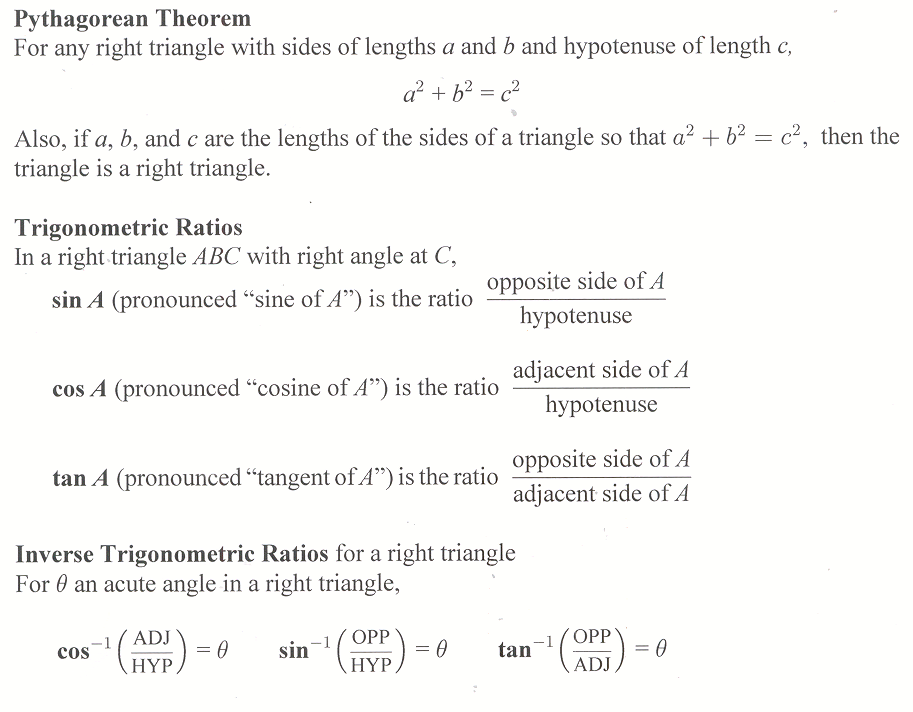## Homework Hints 18-1

### Note: Homework Hints are given only for the Level 1 and Level 2 problems.

However, as you go through the book be sure you look at all the examples in the text. If you need hints for the Level 3 … See the whole entry

## 18.1 Outline

1. What is Calculus?
1. Calculus is the mathematics of motion and change.
2. The three main ideas of calculus are limit, derivative, and integral
1. Achilles and the tortoise
2. limit of a sequence
3. The derivative: the tangent
See the whole entry

## Homework Hints 7-5

### Note: Homework Hints are given only for the Level 1 and Level 2 problems.

However, as you go through the book be sure you look at all the examples in the text. If you need hints for the Level 3 … See the whole entry

## 7.5 Outline

1. Pythagorean theorem
2. Trigonometric ratios
1. sine
2. cosine
3. tangent
3. Inverse trigonometric ratios
1. calculator calculating
2. inverse trigonometric ratios
1. inverse tangent
2. inverse cosine
3. inverse sine
4. Applications
1. angle of elevation
2. angle of depression

## 7.5 Essential IdeasSee the whole entry